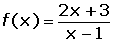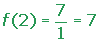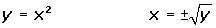Learn from home

The teachersChapters

A function, f, is the inverse of another , f−1, if:

f(a) = b, and f−1(b) = a.Note that:

The domain f−1 is the range of f.

The range of f−1 is the domain of f.

To find the range of a function we have to find the domain of its inverse function.

If two functions are the inverse of each other, their composition is the identity function.

(f o f −1) (x) = (f −1 o f) (x) = x

The graphs of f and f −1 are symmetrical about the bisector of the first and third quadrant.We must distinguish between the inverse function, f−1(x), and the inverse of a function,.

## Example 1.

Write the equation of the function with x and y.

## Example 2.

Work out the value of the variable x as a function of the variable y.

## Example 3.

The variables are exchanged.

Calculate the inverse function of:Check the result for x = 2.Did you like the article?(1 votes, average: 5.00 out of 5)Loading...

Emma

I am passionate about travelling and currently live and work in Paris. I like to spend my time reading, gardening, running, learning languages and exploring new places.

Did you like
this resource?

Bravo!